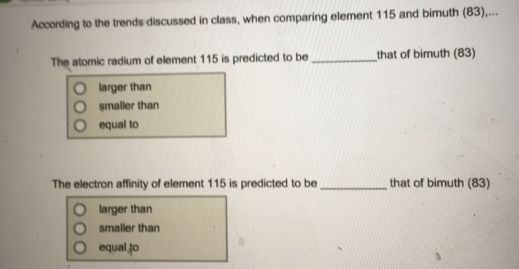# Problem: The atomic radium of element 115 is predicted to be that of bimuth (83) larger than, smaller than, equal to The electron affinity of element 115 is predicted to be that of bimuth (83)larger than, smaller than, equal to

###### FREE Expert Solution

The trend for atomic radius increases from right to left and top to bottom
If moscovium (115) is 1 row below bismuth, Mc has a larger atomic radius than Bi

83% (22 ratings)###### Problem Details

The atomic radium of element 115 is predicted to be that of bimuth (83)

larger than, smaller than, equal to

The electron affinity of element 115 is predicted to be that of bimuth (83)

larger than, smaller than, equal to